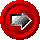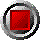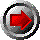GenCurveBondPriceRVOddFirstOAS() functionGenCurveBondPriceRVOddFirstOAS() function

GenCurveBondPriceRVOddFirstOAS(argument list…)

This function returns an OAS given a bond with an odd first coupon (short or long) using a zero curve. The zero curve can be defined either by zero rates or discount factors, and different interpolation methods are offered. The function uses the following arguments:

 Argument Description Restrictions Valuation_Date valuation date (e.g. today) valid Excel date number Settlement_Date bond settlement date valid Excel date number >= Valuation_Date >= Issue_Date Maturity_Date bond maturity date valid Excel date number >= Settlement_Date > Issue_Date > First_Coup_Date Issue_Date the issue date of the bond < First_Coup_Date First_Coup_Date the first coupon date of the bond same coupon cycle as Maturity_Date (i.e. consistent with Freq argument) Coupon annual bond coupon in decimal form (e.g. six percent entered as 0.06). For zero coupon (strip) bonds, enter 0. >= 0 Freq number of bond coupons per annum 1, 2, 4, or 12 DCB day count basis 0 = 30/360 (US) 1 = act/act for US T-Bonds 2 = act/360 3 = act/365 4 = 30/360 (European) 5 = Canadian modified act/365 Redemption redemption value of bond per \$100 par typically \$100 Curve_Type defines how the Zero_Rates array is to be interpreted 0 = continuously compounded riskless rates in, decimal form 1 = discount factors (first must be 1.0, and must be declining) Interpolation the interpolation method to employ for the Zero_Rates array 0 = cubic-spline 1 = linear 2 = log-linear Zero_Dates array of zero coupon curve dates strictly ascending order The first date of this array must be Valuation_Date Zero_Rates an array of zero rates or discount factors corresponding to Zero_Dates Bond_Price the bond price > 0

© 1995-98 Leap of Faith Research Inc.